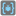• # Did I solve it?

## Question related to missionThe Flat Dictionary

Because this mission does not accept solutions, or allow you to publish them, I thought I would post my solution and see what you think.

```def flatten(dictionary):
stack = [((), dictionary)]
result = {}
while stack:

path, current = stack.pop()
#print(current)
#print(current.items())
for k, v in current.items():
if isinstance(v, dict):

if v == {}:
v = ""
stack.append((path, {k : v}))
continue
stack.append((path + (k,), v))
else:
#print(path, k, v)
result["/".join((path + (k,)))] = v
#print(result)
return result
```

Thanks!7